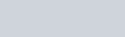Lorem ipsum dolor sit amet, consectetur adipiscing elit, sed do eiusmod tempor incididunt ut labore.

15 St Margarets, NY 10033
(+381) 11 123 4567
ouroffice@aware.com

### KMF#### How the TypeScript Parameters Type Works

The TypeScript `Parameters` Type is used to take the parameters or arguments of a function and create a new type based on them. It is quite useful when we know that the input of a `Function` conforms to a certain type, and we want to replicate that. In this guide, let’s look at how the `Parameters` utility type works.

### TypeScript Custom Types

This guide covers custom types. If you’re new to custom types, read my guide on custom types here.

## How the Parameters Type Works

Imagine you have a function, with a set number of arguments. For example, here is a TypeScript function with two parameters or arguments, `a`, and `b`:

``````const myFunction = (a: string, b: string) => {
return a + b;
}``````

Let’s say we want to run this function. One way is to pass in an array with a tuple type the three dots operator. For example:

``````const myFunction = (a: string, b: string) => {
return a + b;
}

let passArray:[string, string] = [ 'hello ', 'world' ]

// Returns 'hello world'
myFunction(...passArray);``````

Here we define a tuple, which is simply `[string, string]`, which we can pass into myFunction, satisfying both the `a` and `b` arguments.

That works fine, but what if the arguments for `myFunction` change? This is especially likely to happen if `myFunction` is coming from a third-party script. Then we would have to not only update our function but remember to update our tuple type which we pass in. We’d also need to stay up to date with the package `myFunction` is in, in case it changes.

Instead, if we want to ensure they always match, we can use `Parameters` to produce the same type. This will create a tuple type of the arguments, instead of us having to define it manually:

``````type myType = Parameters<typeof myFunction>
// Equivalent to a tuple type of:
// type myType = [ a: string, b: string ]``````

This saves us some hassle in defining custom types since we can now pass anything of the type `myType` into `myFunction` with little fear of error:

``````const myFunction = (a: string, b: string) => {
return a + b;
}
type myType = Parameters<typeof myFunction>
let myArray:myType = [ 'hello ', 'world' ];
myFunction(...myArray)``````

### Typing Specific Parameters With the Parameter Utility Type

Parameter types are also quite flexible, and allow us to define more than just the full set of arguments. For example, if we wanted to only match the type of the first argument in our function `myFunction`, we can reference that like a simple array, by adding ``. The following will match the type of `a`, but if we wanted to match the type of `b` we could use ``:

``````type myType = Parameters<typeof myFunction>
// Equivalent of 'string'``````

That way, we could define custom types for each argument if we have to in our code. For example, here we define two custom types for the first and second parameters of the function, and pass them both into our function:

``````const myFunction = (a: string, b: string) => {
return a + b;
}

type aType = Parameters<typeof myFunction>
type bType = Parameters<typeof myFunction>

let a:aType="hello "
let b:bType="world"

myFunction(a, b)``````

### More Fun With Parameters

Since `Parameters` converts the type of arguments to a new type, we can also pass a function directly into it. The below will produce a type `[ a: string, b: number ]`. This is less useful than taking the parameters from a specific function, but can serve purposes in certain situations:

``type anotherType = Parameters<(a: string, b: number) => void>``